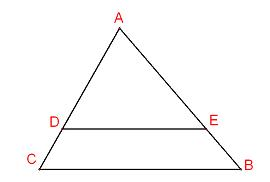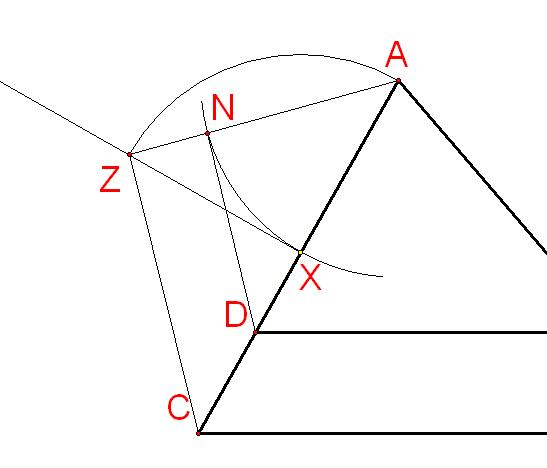#### You may also like### Golden Thoughts

Rectangle PQRS has X and Y on the edges. Triangles PQY, YRX and XSP have equal areas. Prove X and Y divide the sides of PQRS in the golden ratio.### At a Glance

The area of a regular pentagon looks about twice as a big as the pentangle star drawn within it. Is it?### Contact

A circular plate rolls in contact with the sides of a rectangular tray. How much of its circumference comes into contact with the sides of the tray when it rolls around one circuit?

# Half a Triangle

##### Age 14 to 16Challenge Level

Well done Arun from National Public School, Bangalore, India, some quality thinking in devising this solution.

We are given a triangle $ABC$ , and are required to draw a line $DE$ parallel to $CB$ such that it divides the triangle into $2$ of equal areas.The area of triangle $ABC$ is double the area of $AED$.

But, $ADE$ and $ACB$ are similar triangles because $DE$ is parallel to $CB$

We also know that the ratio of the areas of the two similar triangles is equal to the ratio of the squares of corresponding sides.

Which means that the line ratio $AD$:$AC$ must be $1 : \sqrt{2}$
The problem becomes : how to locate $D$ to achieve this ratio.

A square of side length $1$ has a diagonal length of$\sqrt{2}$

or, put another way, an isosceles right-angled triangle has a hypotenuse $\sqrt{2}$ times bigger than the other sides.

Here is a construction to achieve this required ratio.$X$ is any suitable point on $AD$

$ZX$ is perpendicular to $AC$, and $ZX$ is equal in length to $AX$.

So $AXZ$ is an isosceles right-angled triangle.

By sweeping an arc centre $A$ from $X$ to $AZ$ at $N$, $AN$ is made equal to $AX$

$AN$ to $AZ$ is now in the required ratio.

Drawing from $N$ parallel to $ZC$ the point $D$ is reached.

Because $AND$ and $AZC$ are similar triangles, $AD$ and $AC$ are in the required ratio.

Excellent and simple!